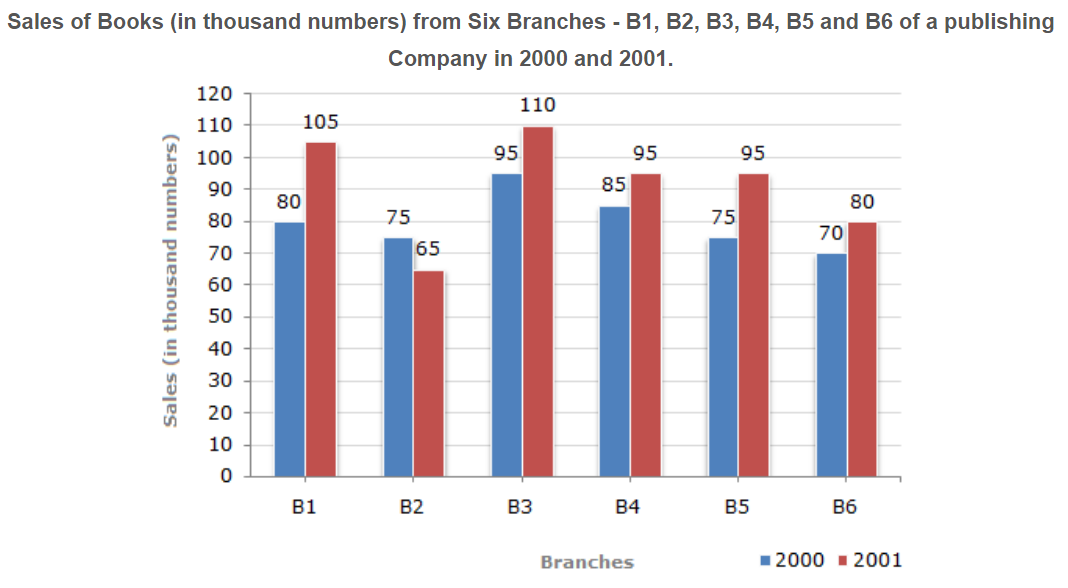# E-PolyLearning

 1. The bar graph given below shows the sales of books (in thousand number) from six branches of a publishing company during two consecutive years 2000 and 2001. What is the ratio of the total sales of branch B2 for both years to the total sales of branch B4 for both years?a. 2:3 b. 3:5 c. 4:5 d. 7:9

 2. The bar graph given below shows the sales of books (in thousand number) from six branches of a publishing company during two consecutive years 2000 and 2001. Total sales of branch B6 for both the years is what percent of the total sales of branches B3 for both the years?a. 68.54% b. 71.11% c. 73.17% d. 75.55%
 3. The bar graph given below shows the sales of books (in thousand number) from six branches of a publishing company during two consecutive years 2000 and 2001. What percent of the average sales of branches B1, B2 and B3 in 2001 is the average sales of branches B1, B3 and B6 in 2000?a. 75% b. 77.5% c. 82.5% d. 87.5%
 4. The bar graph given below shows the sales of books (in thousand number) from six branches of a publishing company during two consecutive years 2000 and 2001. What is the average sales of all the branches (in thousand numbers) for the year 2000?a. 73 b. 80 c. 83 d. 88
 5. The bar graph given below shows the sales of books (in thousand number) from six branches of a publishing company during two consecutive years 2000 and 2001. Total sales of branches B1, B3 and B5 together for both the years (in thousand numbers) is?a. 250 b. 310 c. 435 d. 560